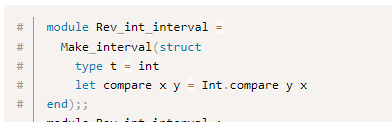# How to write abstraction in Rescript

I know that we can use module type as an interface, but i still feel not very normal with using it. Do we have or consider making a way to write interfaces? Just like the trait in Rust.

I have made a simple abstraction example, there are some boilerplates in my code. Could someone make it more simpler? Also, can we define anomous module in Rescript like we do it in OCaml?Below is just a simple example.

``````module type Handle = {
type t
let width: t => int
let height: t => int
}

module MakeSizeHandle = (Handle: Handle) => {
type t = Handle.t
@inline
let getSize = t => {
(t->Handle.width, t->Handle.height)
}
}

module Gif = {
type t = {
width: int,
height: int,
}
module Handle = {
type t = t
let width = (t: t) => t.width
let height = (t: t) => t.height
}
module GetGifSize = MakeSizeHandle(Handle)
}

module Picture = {
type t = {
width: int,
height: float,
}
module Handle = {
type t = t
let width = (t: t) => t.width
let height = (t: t) => t.height->Js.Math.unsafe_round
}
module GetPictureSize = MakeSizeHandle(Handle)
}

module Jpg = {
type t = {
width: int,
height: string,
}
module Handle = {
type t = t
let width = t => t.width
let height = t =>
t.height
->Belt.Int.fromString
->(
v => {
switch v {
| Some(v) => v
| None => Js.Exn.raiseError(`error parsing height of Jpg`)
}
}
)
}

module GetJpgSize = MakeSizeHandle(Handle)
}

module Material = {
type t = Gif(Gif.t) | Picture(Picture.t) | Jpg(Jpg.t)
let handle = material => {
switch material {
| Gif(gif) => gif->Gif.GetGifSize.getSize
| Picture(picture) => picture->Picture.GetPictureSize.getSize
| Jpg(jpg) => jpg->Jpg.GetJpgSize.getSize
}->(size => size->Js.log2(`size of material: `, _))
}
}

Material.Gif({
width: 3,
height: 4,
})->Material.handle

Material.Picture({
width: 3,
height: 5.,
})->Material.handle

Material.Jpg({
width: 3,
height: "8",
})->Material.handle

``````

Seems like you’re trying to reach for a form of polymorphism? I think a more idiomatic alternative is to make use of variant types and pattern match over them

``````type material =
| Gif(int, int)
| Picture(int, float)
| Jpg(int, string)

let parseStringDimension = dimension =>
switch dimension->Belt.Int.fromString {
| Some(v) => v
| None => {
Js.Console.error(`Error parsing height of Jpg ('\${dimension}')`)
0
}
}

let getSize = material => switch material {
| Gif(w, h) => (w, h)
| Picture(w, h) => (w, Js.Math.unsafe_round(h))
| Jpg(w, h) => (w, parseStringDimension(h))
}

let handle = material => {
let size = material->getSize
Js.Console.log2(`Size of the material: `, size)
}

let gif = Gif(3, 4)
handle(gif)

let pic = Picture(3, 5.)
handle(pic)

let jpg = Jpg(3, "8")
handle(jpg)
``````

No, using pattern match and writting size extraction in every arm may be very appropriate for this simple example. But this is just a special example i made for abstraction, the real use case may be much complicated and repetetive, just like writting a sort method for a generic array. If i write this example code or write a generic sort function in Rust, i would just define a trait first, implement the trait for structs i want to use, and then just write a function which receives a parameter which implements the trait. This is much simple and idiomatic. While in Rescript, we need to define a lot of modules to achieve the same result.

Yes, you could do something like:

``````module Gif = {
type t = {
width: int,
height: int,
}
module GetGifSize = MakeSizeHandle({
type t = t
let width = (t: t) => t.width
let height = (t: t) => t.height
})
}
``````
1 Like